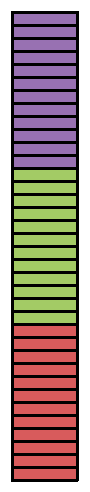### Home > CC1MN > Chapter 9 Unit 9 > Lesson CC1: 9.2.1 > Problem9-38

9-38.

Express each of the following lengths in feet.

Remember that $12$ inches are equal to $1$ foot and $3$ feet are equal to $1$ yard. These conversions will come in handy!

1. $24$ inches

1. $18$ inches

1. $9$ inches

Try drawing a diagram of how many inches are in a foot to compare to each of these measures.

For instance, James converted 36 inches to feet by drawing the diagram at right. He could see that there are 3 groups of 12 inches in 36 inches, so that means 36 inches is equal to 3 feet!

1. $2$ yards

1. $3$ inches

1. $2\frac{1}{2}$ yards

If one yard is 3 feet, how many feet are in 2 yards? Can you use multiplication? Why would that make sense?

$3\text{ inches }=\frac{1}{4}\text{ feet.}$

Don't forget to show your work.$2\frac{1}{2}\text{ yards }=7\frac{1}{2}\text{ feet.}$